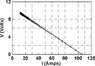# Variable resistor graph

• mitsuruangel
In summary, the conversation discusses a circuit with a variable resistor connected to a battery, and the graph shows data collected as the resistor is varied. The questions asked are what the voltage, V0, is and what the internal resistance, r, is. The conversation also includes a question about where V0 should be measured and an explanation of why it should be measured at the lowest current. The conversation concludes with a discussion about the special properties of the point where the graph cuts the y axis.

## Homework Statement

A variable resistor, R, is connected to the terminals of a battery (dashed box in the diagram). The graph below shows data collected in the circuit as R is varied. What is V0, in volts?

What is the internal resistance, r, in ohms?

should the V0 be measured at the top of the line where it is at the smallest current? because that is what the TA explained to do, I tried and I can't seem to get it right

#### Attachments

•Untitled2.jpg
26.2 KB · Views: 921
Did you extend the line backwards to where I=0(where it cuts the y axis)

mitsuruangel said:

## Homework Statement

A variable resistor, R, is connected to the terminals of a battery (dashed box in the diagram). The graph below shows data collected in the circuit as R is varied. What is V0, in volts?

What is the internal resistance, r, in ohms?

should the V0 be measured at the top of the line where it is at the smallest current? because that is what the TA explained to do, I tried and I can't seem to get it right

And adding one more question to Dadface's post, what is special about where it cuts the y axis?

that worked! thanks

mitsuruangel said:
that worked! thanks

But why did it work. Do you understand that part?

Because V0 should be at lowest current (ie when it is 0) but the internal resistance keeps it from actually getting there?

mitsuruangel said:
Because V0 should be at lowest current (ie when it is 0)

That part is correct. V0 is the "open circuit voltage", so when no current is flowing. The other thing you can use to figure out the internal resistance is the "short circuit current". Once you have V0 and Rs, you have characterized your voltage source.